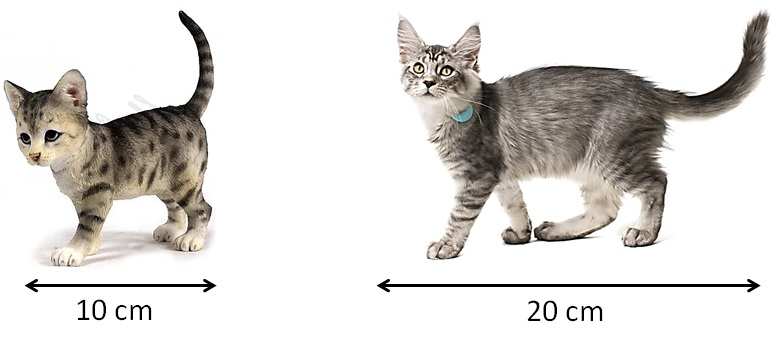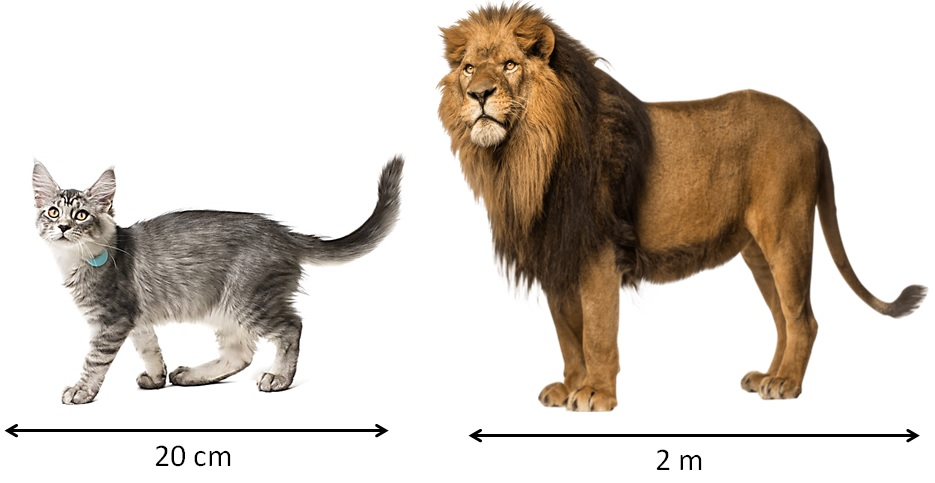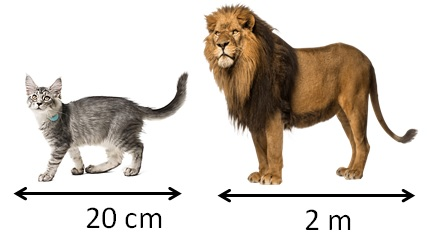Finding ratio by changing units

Chapter 12 Class 6 Ratio and Proportion
Concept wise

Suppose I have two catsSo, Ratio of length of cat to baby cat = 20/10

= 2/1

= 2 : 1

So, cat is twice as long as baby cat

Now, let’s takeSo, Ratio of length of the cat to the Lion = 20/2

= 10/1

= 10 : 1

So, cat is 10 times as long as Lion?

WRONG

Here units are different

Cat’s length is in cm

Lion’s length is in m

So, we convert cm to m or cmSo,

Cat’s length = 20 cm

Lion’s length = 2 m

= 2 × 100 cm

= 200 cm

So, Ratio of cat’s length to lion’s length = 20/200

= 1/10

= 1 : 10

So, Cat’s length is 1/10 th of lion’s

So, we have to keep in mind units when forming ratio

Learn in your speed, with individual attention - Teachoo Maths 1-on-1 Class